## How many grams of Ca(OH)2 would be needed to make 500 mL of a 0.450 mol/L solution? 113 g 133 g 41.2 g 16.68 g

Question

How many grams of Ca(OH)2 would be needed to make 500 mL of a 0.450 mol/L solution?

113 g
133 g
41.2 g
16.68 g

in progress 0
2 months 2021-08-01T19:10:31+00:00 1 Answers 2 views 0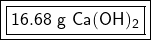Explanation:

Molarity is a measure of concentration in moles per liter.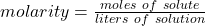The molarity is 0.450 moles per liter. The moles of solute is unknown.

The volume of the solution is 500 milliliters, but we want to know the liters. We need to convert the units. Remember that 1 liter contains 1000 milliliters.

•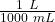•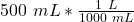•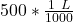•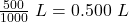So, we have values for all the variables:

• molarity= 0.450 mol/L
• moles of solute = x
• liters of solution =0.500 L

Substitute the values into the formula.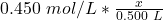We want to solve for x, the moles of solute. x is being divided by 0.500 liters. The inverse of division is multiplication, so multiply both sides by 0.500 L.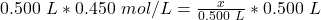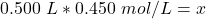The units of liters cancel.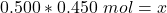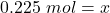Now we have the moles and need to convert to grams. This is done using the molar mass (mass per 1 mole). The values are found on the Periodic Table as atomic masses, but the units are grams per mole instead of atomic mass units.

The solute is Ca(OH)₂, so lookup the molar masses of the individual elements.

• Ca:40.08 g/mol
• O: 15.999 g/mol
• H: 1.008 g/mol

Note there is a subscript of around OH. There are 2 hydroxide molecules, so there are 2 oxygen and 2 hydrogen atoms. We must multiply their molar masses by 2 before adding all the values.

• Ca(OH)₂= 40.08 + 2(15.999)+2(1.008)=74.094 g/mol

Use the molar mass as a ratio.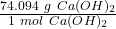Multiply by the number of moles calculated.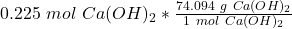The units of moles of calcium hydroxide cancel.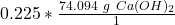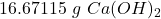16.68 grams of calcium hydroxide are needed.